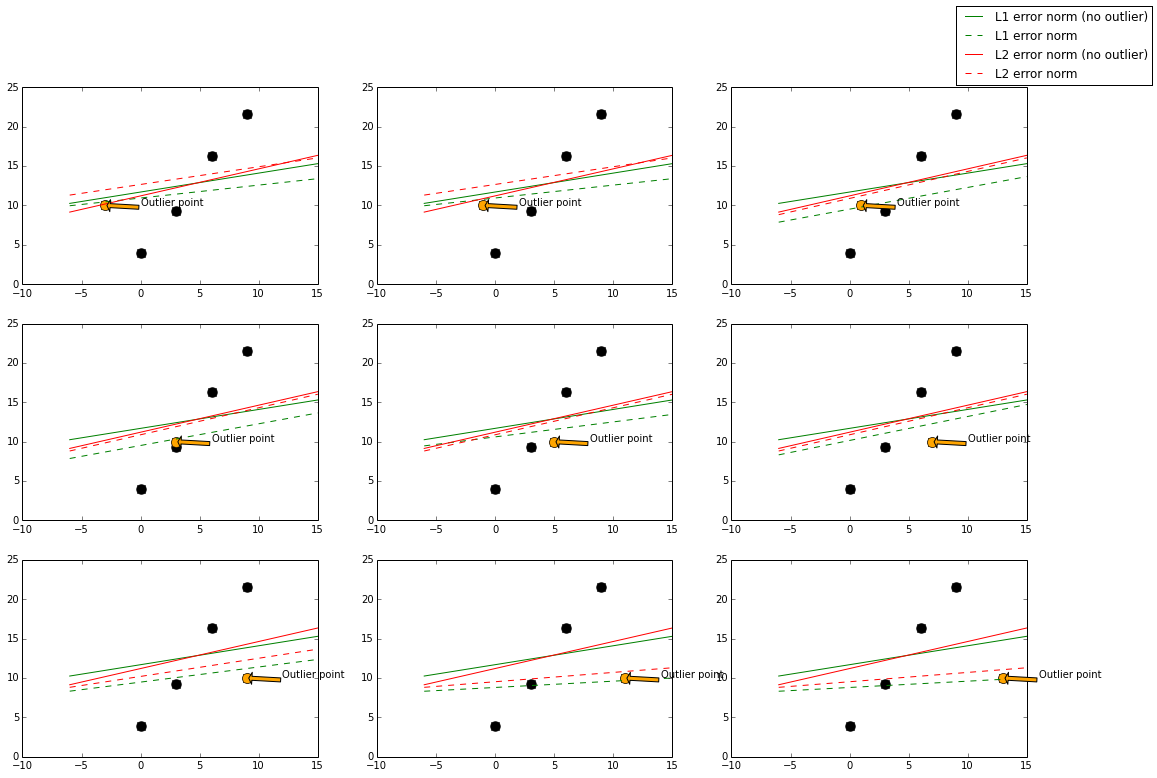In :
print \
"""
Author: log0 <[email protected]>
Date: 2014/11/30
Website: http://www.chioka.in

Script to validate visually the stability property of L1-norm and L2-norm loss function.

Experiment Design:
- Generate N basic points at with varying y = b * x + c + random_number.
- Generate M datasets with an outlier point clearly outside this range.
- Plot M graphs to see how different outlier points will cause the different model to behave.
"""

Author: log0 <[email protected]>
Date: 2014/11/30
Website: http://www.chioka.in

Script to validate visually the stability property of L1-norm and L2-norm loss function.

Experiment Design:
- Generate N basic points at with varying y = b * x + c + random_number.
- Generate M datasets with an outlier point clearly outside this range.
- Plot M graphs to see how different outlier points will cause the different model to behave.


In :
import numpy as np
import matplotlib.pyplot as plt
import random
from sklearn.linear_model import *
from sklearn.ensemble import *

In :
# Set inline plots in IPython Notebooks
%matplotlib inline
plt.rcParams['figure.figsize'] = (18, 12) # Set default figure size of plots

In :
def linear_point(x, b, c, random_scale = 1):
return b * x + c + random.random() * random_scale * random.choice([-1, 1])

In :
# Fixed y, because we want to see how an outlier point affects the model.
def outlier_point(x, b, c, random_scale = 1):
return 10

In :
# Specifies to generate 3 x 3 subplots.
n_plots_x = 3
n_plots_y = 3

# Generates data points from [0,n) with x_step_size = 3.
n = 10
x_step_size = 3
# b and c are parameters of the linear function that generates the data points.
b = 2
c = 5
# Adds noise to the generated data points.
random_scale = 1 * b
base_x = np.arange(0, n, x_step_size)
base_y = [linear_point(x, b, c, random_scale) for x in base_x]

# Generates the set of outlier points.
outlier_x = np.linspace(-x_step_size, n + x_step_size, n_plots_x * n_plots_y)
outlier_y = [outlier_point(x, b, c) for x in outlier_x]

In :
# Generates the subplots, and reshape it so we can iterate over with an index.
figure, axes_list = plt.subplots(n_plots_x, n_plots_y)
axes_list = axes_list.reshape((n_plots_x * n_plots_y))

# This is just for plotting purposes, so is not important.
min_x = base_x - x_step_size * 2
max_x = base_x[-1] + x_step_size * 2

# This for loop generates a single plot for each outlier point we have. Each outlier point
# is concatenated with the non-outlier points (base_x, base_y), so effectively we will have
# different datasets which includes one single outlier point.
#
# Then, we train the L1-norm and L2-norm models on both the set of points with outliers and
# without outliers, just to see how the different models behave to outliers and without outliers.
for index in xrange(outlier_x.shape):
# Get the right subplot.
axes = axes_list[index]

# mixed_x and mixed_y is a training dataset that includes also the outlier point.
mixed_x = np.hstack(([outlier_x[index]], base_x))
# Sort and get indices so we plot correctly.
mixed_x_indices = np.argsort(mixed_x)
mixed_x = mixed_x[mixed_x_indices]
mixed_x = mixed_x.reshape((mixed_x.shape, 1))  # Reshape to have N+1 examples
mixed_y = np.hstack(([outlier_y[index]], base_y))
# Sort by the x indices so y values align with the x values.
mixed_y = mixed_y[mixed_x_indices]

base_x_points = base_x.reshape((base_x.shape, 1))

# Trains a L1-norm model without the outlier point. Act as control to see how the
# L1-norm model shifts.
base_l1_clf.fit(base_x_points, base_y)
base_l1_fitted_x = np.array([min_x, max_x])
base_l1_fitted_y = [base_l1_clf.predict(x) for x in base_l1_fitted_x]

# Trains a L2-norm model without the outlier point. Act as control to see how the
# L2-norm model shifts.
base_l2_clf = GradientBoostingRegressor(n_estimators = 5, loss = 'ls')
base_l2_clf.fit(base_x_points, base_y)
base_l2_fitted_x = np.array([min_x, max_x])
base_l2_fitted_y = [base_l2_clf.predict(x) for x in base_l2_fitted_x]

# Trains a L1-norm model with the outlier point. We will compare this with base_l1_clf
# to see how much change it deviates.
l1_clf.fit(mixed_x, mixed_y)
l1_fitted_x = np.array([min_x, max_x])
l1_fitted_y = [l1_clf.predict(x) for x in l1_fitted_x]

# Trains a L2-norm model with the outlier point. We will compare this with base_l2_clf
# to see how much change it deviates.
l2_clf = GradientBoostingRegressor(n_estimators = 5, loss = 'ls')
l2_clf.fit(mixed_x, mixed_y)
l2_fitted_x = np.array([min_x, max_x])
l2_fitted_y = [l2_clf.predict(x) for x in l2_fitted_x]

# Plots the base points which has noise but no outliers.
axes.plot(mixed_x, mixed_y, marker = 'o', markersize = 10, linestyle = '', color = 'black')
# Plots the outlier point, and annotate it.
axes.plot(outlier_x[index], outlier_y[index], 'o', markersize = 10, markerfacecolor = 'orange', linestyle = '')
axes.annotate('Outlier point', xy = (outlier_x[index], outlier_y[index]), xytext = (outlier_x[index] + 3, outlier_y[index]), arrowprops = dict(facecolor='orange', shrink=0.05))
# Plots the fitted lines.
base_l1_line = axes.plot(base_l1_fitted_x, base_l1_fitted_y, marker = '', markersize = 10, linestyle = '-', color = 'green', label = 'L1 error norm (no outlier)')
l1_line = axes.plot(l1_fitted_x, l1_fitted_y, marker = '', markersize = 10, linestyle = '--', color = 'green', label = 'L1 error norm')
base_l2_line = axes.plot(base_l2_fitted_x, base_l2_fitted_y, marker = '', markersize = 10, linestyle = '-', color = 'red', label = 'L2 error norm (no outlier)')
l2_line = axes.plot(l2_fitted_x, l2_fitted_y, marker = '', markersize = 10, linestyle = '--', color = 'red', label = 'L2 error norm')
figure.legend((base_l1_line, l1_line, base_l2_line, l2_line), ('L1 error norm (no outlier)', 'L1 error norm', 'L2 error norm (no outlier)', 'L2 error norm'), 'upper right')In :


In :


In [ ]: